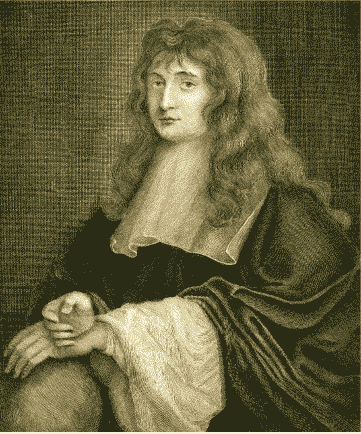## Assumed knowledge

The content of the modules:

## Motivation

I do not define time, space, place, and motion, as being well known to all. Only I must observe, that the common people conceive those quantities under no other notions but from the relation they bear to sensible objects. And thence arise certain prejudices, for the removing of which it will be convenient to distinguish them into absolute and relative, true and apparent, mathematical and common.

— Isaac Newton (1642–1727)

Mathematics is now applied to all branches of science, engineering, commerce and the social sciences. But traditionally, mathematics was applied to the study of science, and principally to physics. The study of physics depends on mathematical methods and models, particularly calculus and differential equations.

From the 17th century onwards, mathematics developed in two directions: pure and applied. One of the first areas of applied mathematics to be studied in the 17th century was motion in a straight line, often referred to as the kinematics of a particle moving in a straight line.

Sir Isaac Newton first developed both the differential and integral calculus in the 1660s, then shortly afterwards turned his attention to kinematics. In his 1671 work Treatise on methods, Newton listed the two central problems of calculus as:

• 'Given the length of the space continuously (that is, at every time), to find the speed of the motion at any time proposed.' (In other words, find the speed of a particle given the position.)
• 'Given the speed of motion continuously, to find the length of the space described at any time proposed.' (In other words, find the position given the speed.)

These problems were the motivation for Newton's work on calculus.

In this module, we deal with the quantities: position, displacement, distance, velocity, speed, acceleration and time. We establish mathematical models for the motion of a particle in a straight line. Initially, we deal with the special case of constant acceleration.

The study of motion in a straight line should be included in any introductory course in calculus. It serves both as a context for understanding the basic concepts of calculus and as an important application of calculus.Portrait of Isaac Newton when undertaking a B.A. at Trinity College, 1677. Engraved by Burnet Reading (1749/50–1838) from a head painted by Sir Peter Lely (1618–1680), published 1799. Source: University of Cambridge, Institute of Astronomy Library.

Next page - Content - Position, displacement and distance